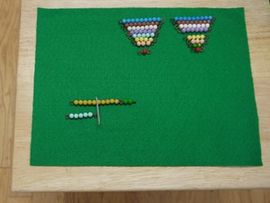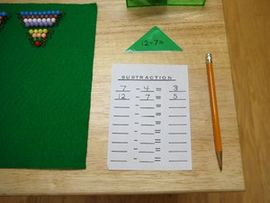# Subtraction With Bead Bars

 We've updated our prices on products from many online Montessori stores! If we've missed your favorite store, please let us know!
Subtraction With Bead Bars
Math - Memory Work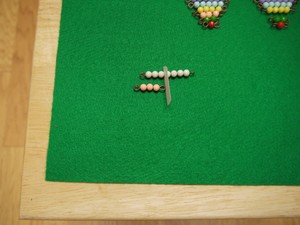LevelPrimary
Age4 - 5
Materialstwo bead stairs (1-10 and 1-9)
pointer for counting
subtraction problem slips
paper
pencil
table-mat (if at table)

This activity allows the child to figure out subtraction facts.

## Presentations

1. Take the material to a mat or table. (If at a table, you will need a table-mat.)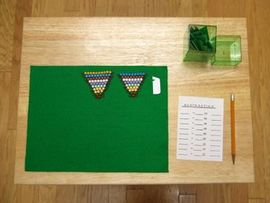2. Draw a problem slip and copy it to the paper.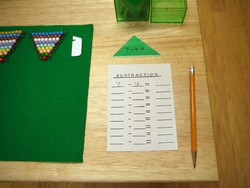3. Take the bead bar for the first number in the problem (the minuend) from the first bead stair.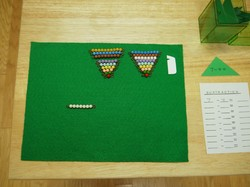4. Count off with the pointer the second number in the problem (the subtrahend) starting from the right.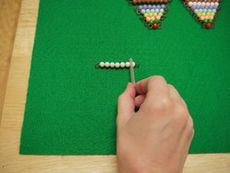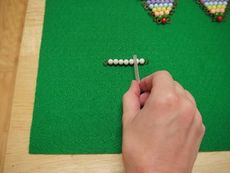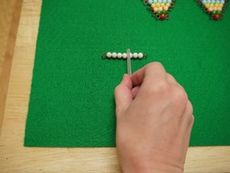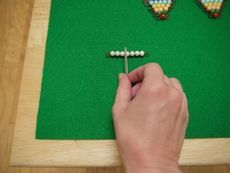5. Leave the counter in place and point with a finger to count the remaining beads.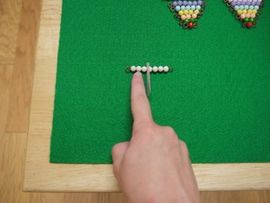6. Take a bead bar from the second bead stair that matches up with the number of remaining beads. This is the answer. Write the answer on the paper.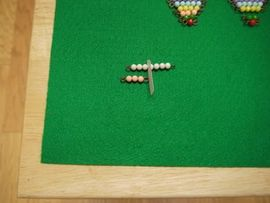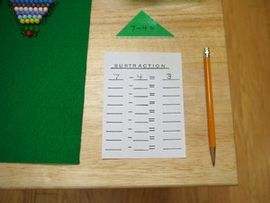7. For problems in which the first number is greater than ten start by writing the problem on the paper.8. Then lay out the first number as a combination of a ten bar and the appropriate smaller bead bar.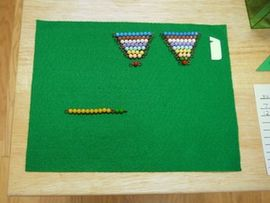9. Count from the right as before, then leave the counter in place and count the remaining beads with your finger.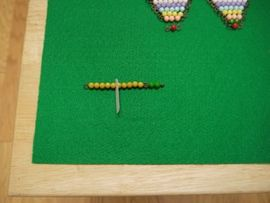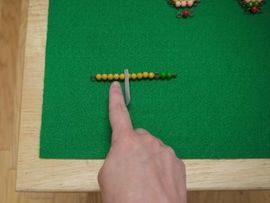10. Find the bead bar for the answer from the second bead stair. Record the answer on the paper.